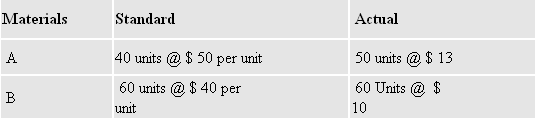222

## Latest \$type=blogging\$count=5\$author=hide\$comment=hide\$label=hide\$date=hide\$show=home

For explaining the steps of calculating material mix variance, we are taking following examples:

1. When there is no difference between actual and standard weight of material:

Calculate Material Mix Variance. Following information are given:

 Materials Standard Actual A 200 units @ \$ 12 160 units @ \$ 13 B 100 units @ \$ 10 140 Units @  \$ 10

Both Material A and Material B's Standard weight and actual weight is 300 units.

Due to shortage of material A, it was decided to reduce the consumption of A by 15% and increase that of material B by 30%.

Material Mix Variance (Formula) = Standard Unit Cost ( Revised Standard Quantity - Actual Quantity)

Important Steps : For calculating of material mix variance, we need to calculate revised standard quantity

Revised standard Quantity of A material = Actual Quantity of A - % of reduction

= 200 units - 200 X 15% = 170 Units

Revised Standard Quantity of B Material = Actual Quantity of B + % of Increase

= 100 units + 100 X 30% = 130 units

Material Mix Variance of A Material = \$ 12 ( 170 - 160 ) = \$ 120 ( Favourable because actual quantity is less than revised standard quantity)

Material Mix Variance of B Material = \$ 10 ( 130 - 140 ) = \$ 100 ( Unfavourable because actual is more than revised standard quantity)

--------------------------------------------------------------------------------
Total of Material Mix Variance = = \$ 20 ( Favourable)

======================================================

2. When Both Actual and standard weight of materials are different :

From the data given below, calculate the material mix variance

Standard weight of material A and b is 100 which is different from actual weight of material A and B which is 110 units.

Material Mix Variance ( Formula) = { Total weight of actual mix/ total weight of standard mix ) X Standard cost of standard mix } - Standard cost of actual mix

Important steps : First, calculate

Total weight of actual mix = 50 units of A material + 60 units of B material = 110 units

Total weight of standard mix = 40 units of A material + 60 units of B material = 100 units

Put the value in the formula

MMV = { 110/100 X ( 40 units X \$ 50 + 60 units X \$ 40 ) } - ( \$ 50 X 50 units + \$ 40 X 60 units )

\$ 4840 -\$ 4900 = \$ 60 UnfavourableName

ltr
item
Accounting Education: How to Calculate Material Mix Variance
How to Calculate Material Mix Variance
http://3.bp.blogspot.com/-4AcyhKwCo2U/TfbrxASp8fI/AAAAAAAAGoE/1Ngy_7jAR6M/s1600/mix.PNG
http://3.bp.blogspot.com/-4AcyhKwCo2U/TfbrxASp8fI/AAAAAAAAGoE/1Ngy_7jAR6M/s72-c/mix.PNG
Accounting Education
https://www.svtuition.org/2011/06/how-to-calculate-material-mix-variance.html
https://www.svtuition.org/
https://www.svtuition.org/
https://www.svtuition.org/2011/06/how-to-calculate-material-mix-variance.html
true
2410664366776677676
UTF-8